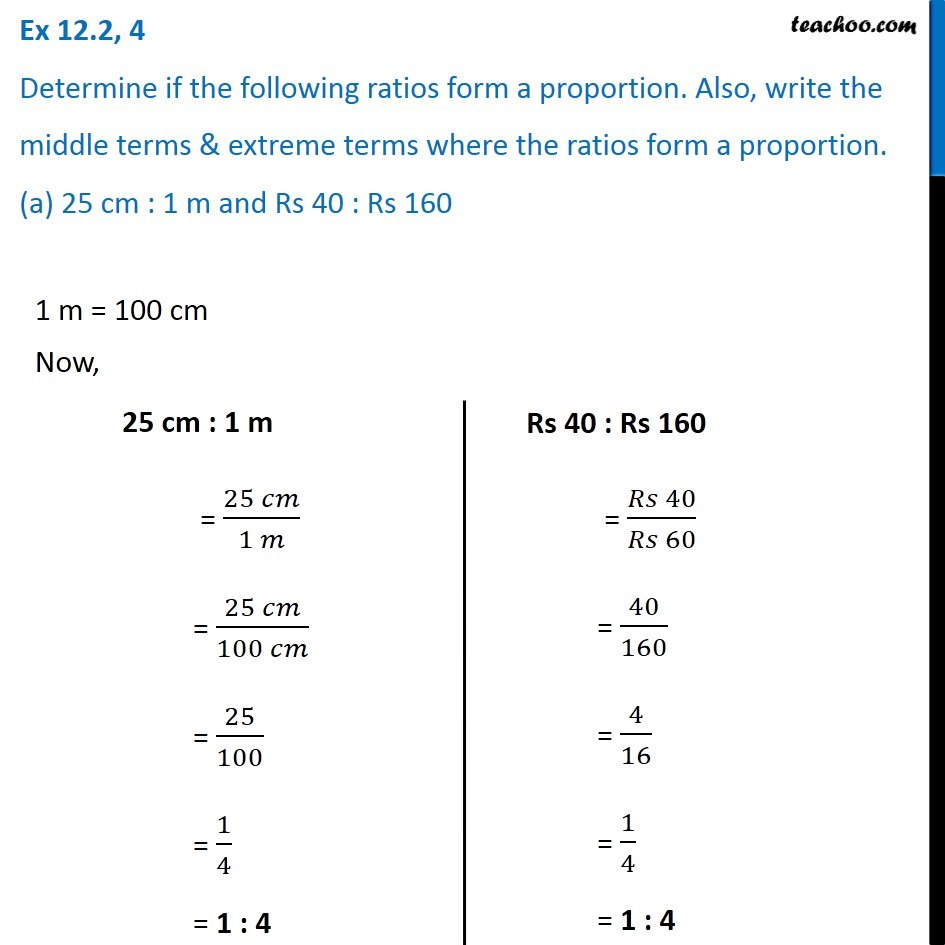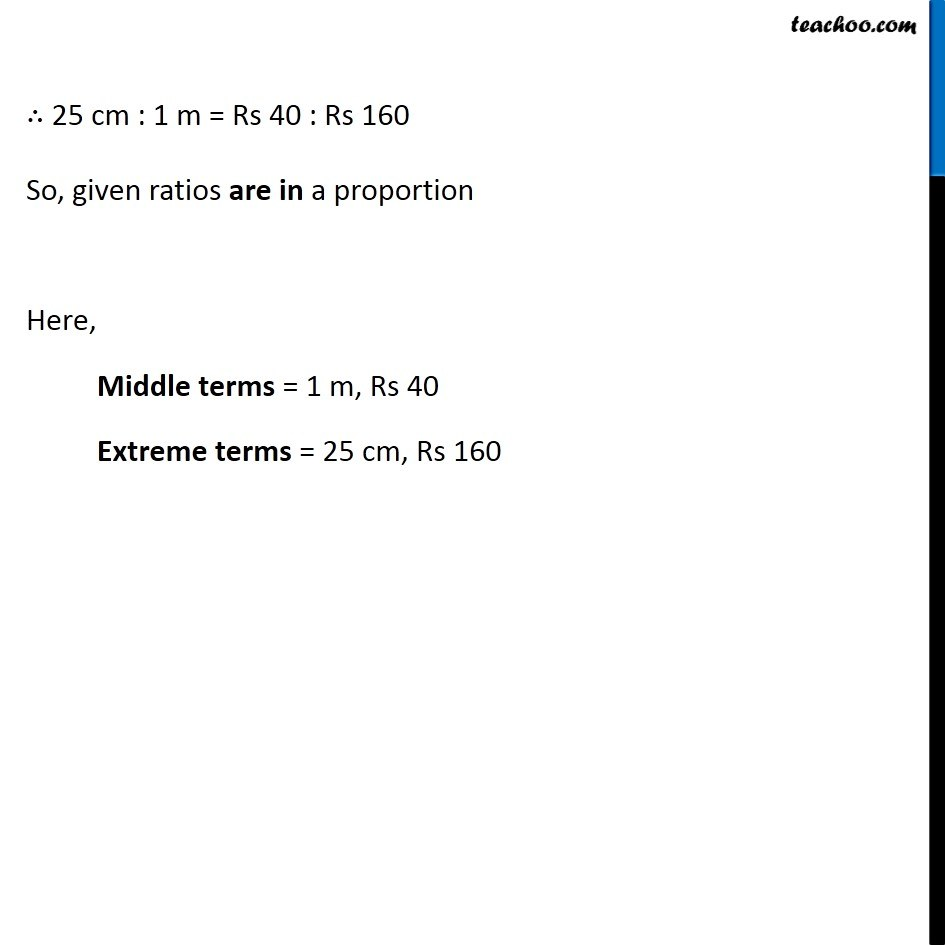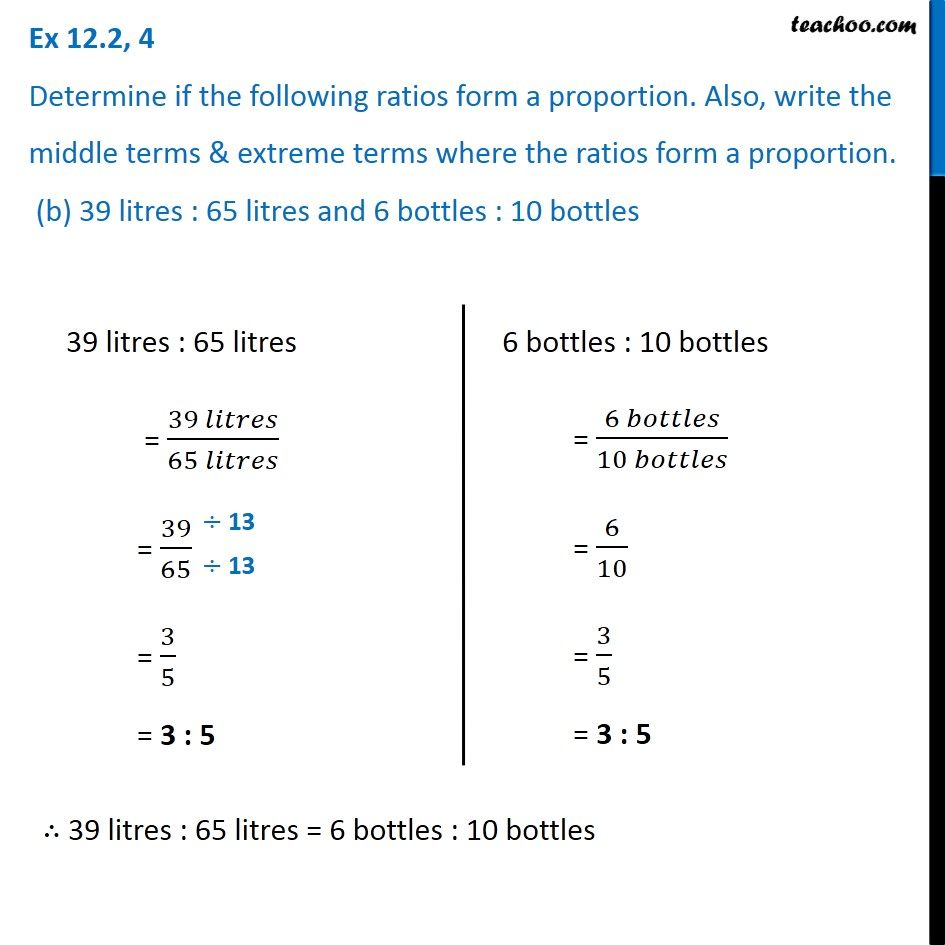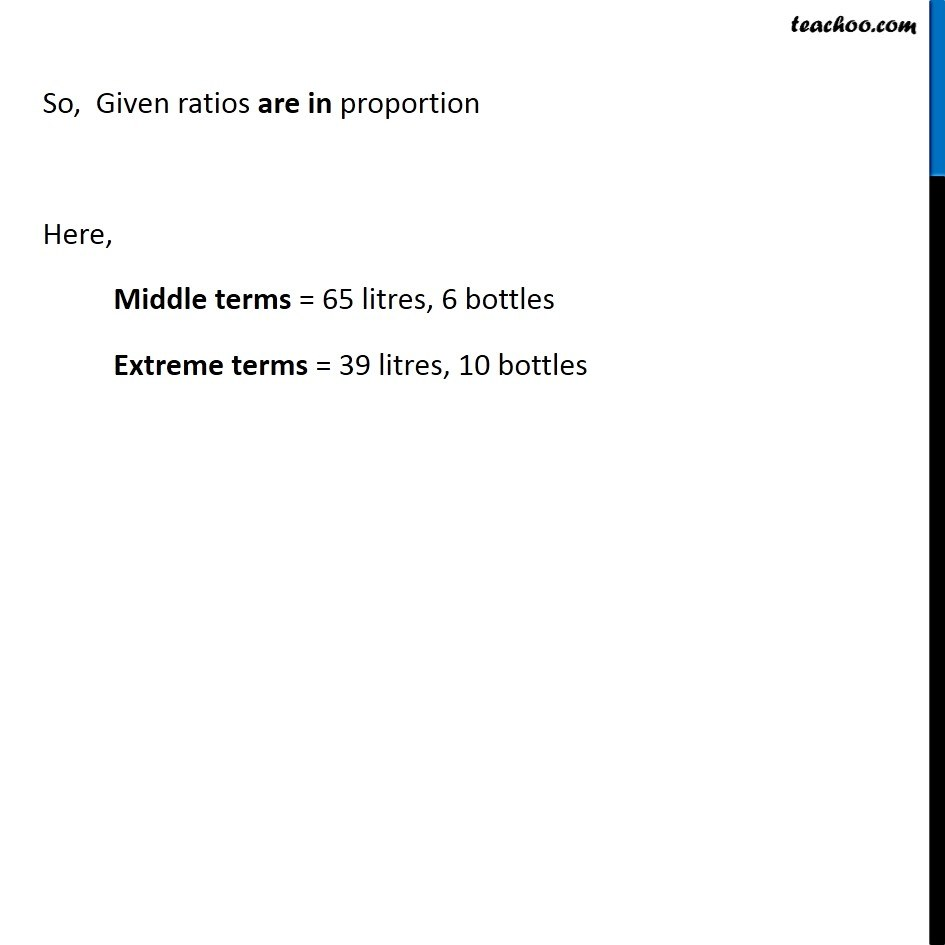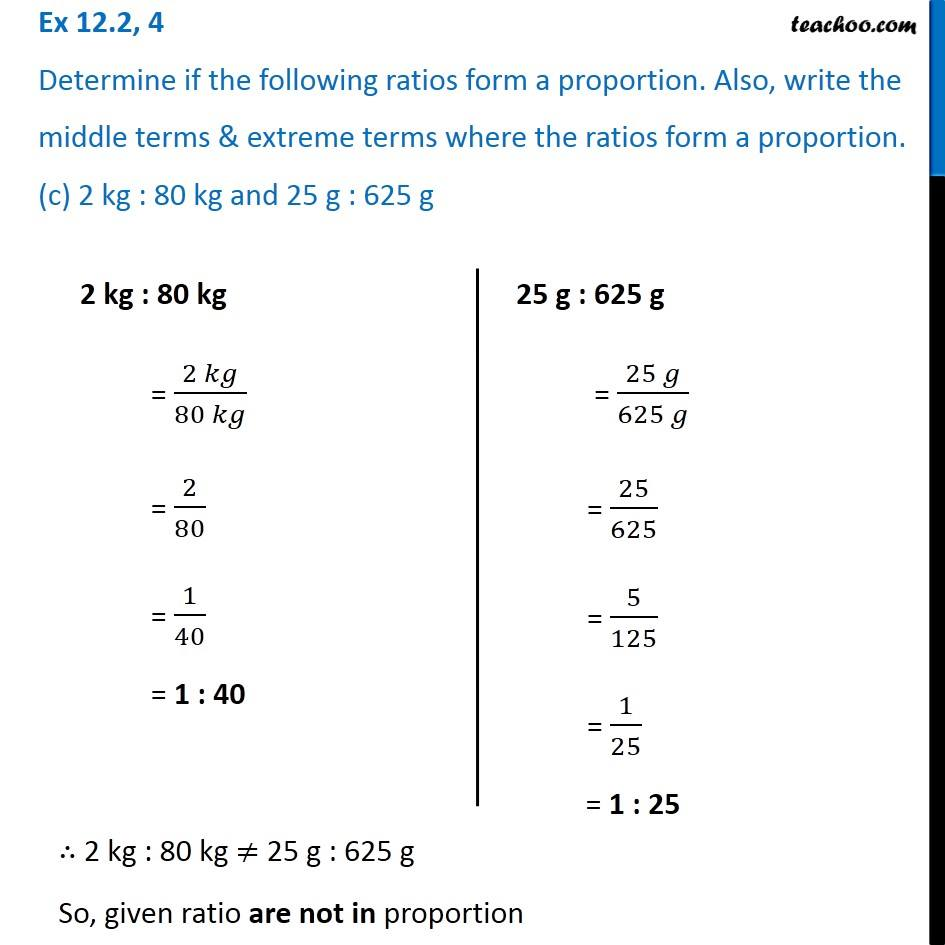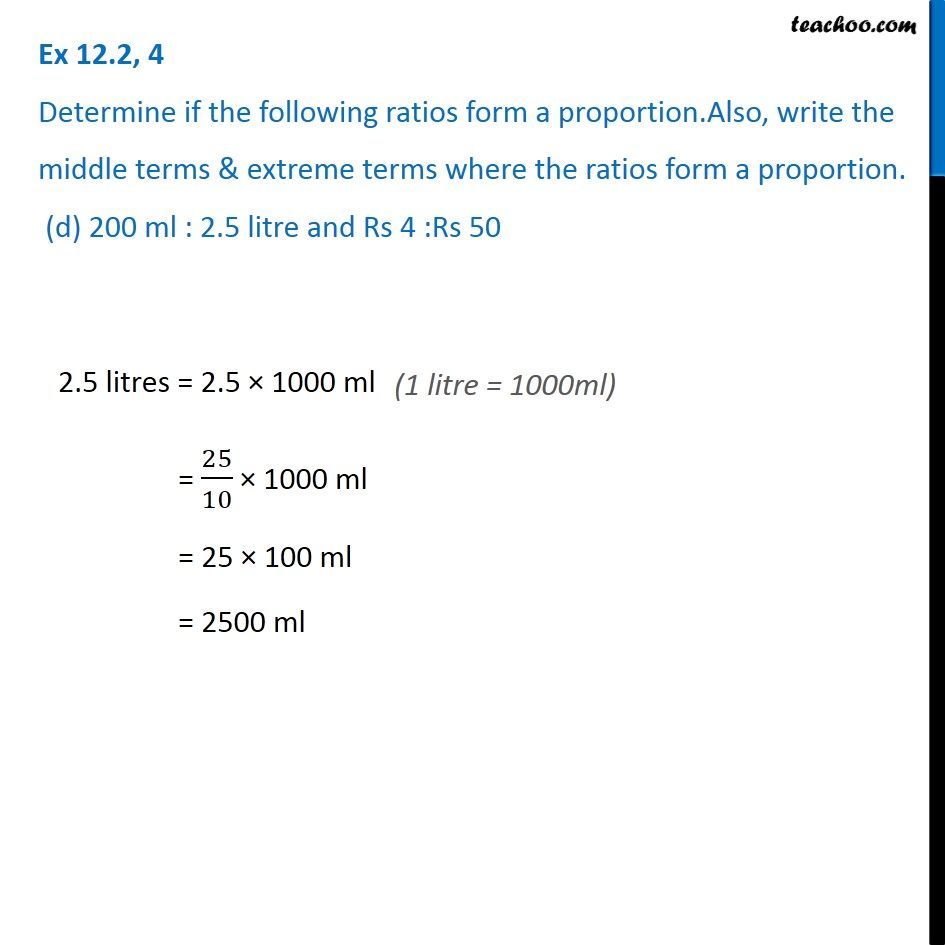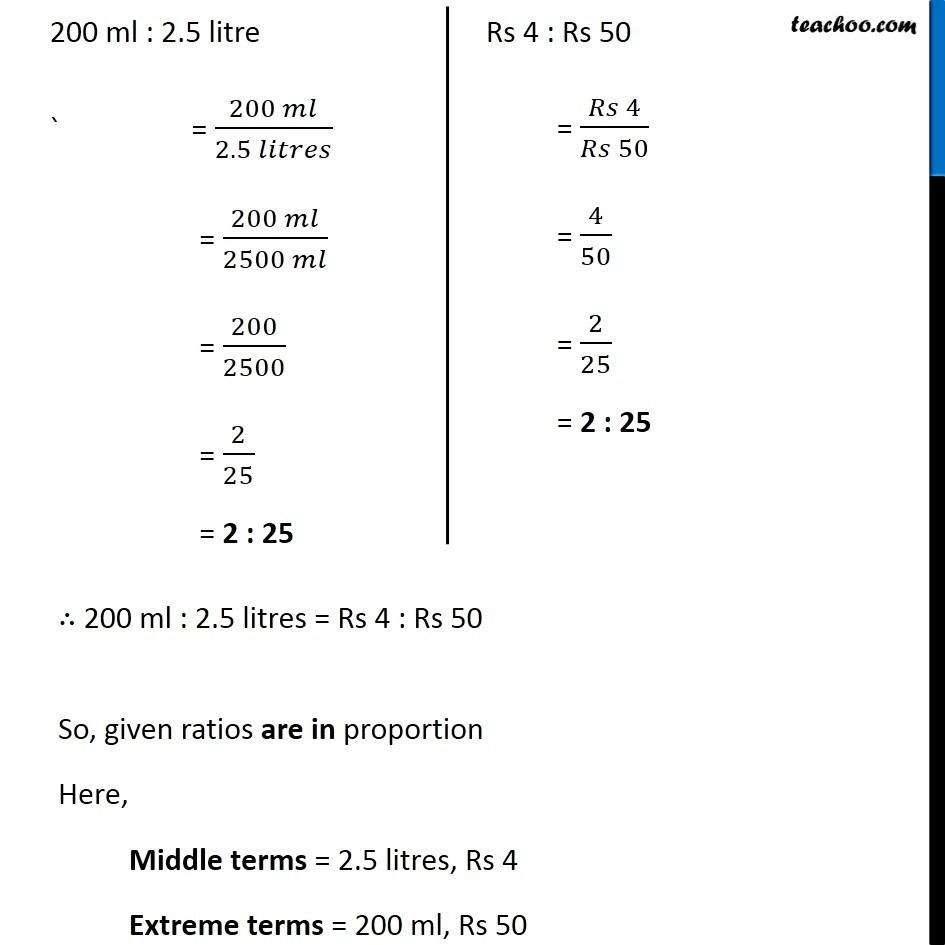Subscribe to our Youtube Channel - https://you.tube/teachoo

1. Chapter 12 Class 6 Ratio And Proportion
2. Serial order wise
3. Ex 12.2

Transcript

Ex 12.2, 4 Determine if the following ratios form a proportion. Also, write the middle terms & extreme terms where the ratios form a proportion. (a) 25 cm : 1 m and Rs 40 : Rs 160 1 m = 100 cm Now, 25 cm : 1 m = (25 𝑐𝑚)/(1 𝑚) = (25 𝑐𝑚)/(100 𝑐𝑚) = 25/100 = 1/4 = 1 : 4 Rs 40 : Rs 160 = (𝑅𝑠 40)/(𝑅𝑠 60) = 40/160 = 4/16 = 1/4 = 1 : 4 ∴ 25 cm : 1 m = Rs 40 : Rs 160 So, given ratios are in a proportion Here, Middle terms = 1 m, Rs 40 Extreme terms = 25 cm, Rs 160 Ex 12.2, 4 Determine if the following ratios form a proportion. Also, write the middle terms & extreme terms where the ratios form a proportion. (b) 39 litres : 65 litres and 6 bottles : 10 bottles39 litres : 65 litres = (39 𝑙𝑖𝑡𝑟𝑒𝑠)/(65 𝑙𝑖𝑡𝑟𝑒𝑠) = 39/65 = 3/5 = 3 : 5 6 bottles : 10 bottles = (6 𝑏𝑜𝑡𝑡𝑙𝑒𝑠)/(10 𝑏𝑜𝑡𝑡𝑙𝑒𝑠) = 6/10 = 3/5 = 3 : 5 ∴ 39 litres : 65 litres = 6 bottles : 10 bottles So, Given ratios are in proportion Here, Middle terms = 65 litres, 6 bottles Extreme terms = 39 litres, 10 bottles Ex 12.2, 4 Determine if the following ratios form a proportion. Also, write the middle terms & extreme terms where the ratios form a proportion. (c) 2 kg : 80 kg and 25 g : 625 g2 kg : 80 kg = (2 𝑘𝑔)/(80 𝑘𝑔) = 2/80 = 1/40 = 1 : 40 25 g : 625 g = (25 𝑔)/(625 𝑔) = 25/625 = 5/125 = 1/25 = 1 : 25 ∴ 2 kg : 80 kg ≠ 25 g : 625 g So, given ratio are not in proportion Ex 12.2, 4 Determine if the following ratios form a proportion.Also, write the middle terms & extreme terms where the ratios form a proportion. (d) 200 ml : 2.5 litre and Rs 4 :Rs 50 2.5 litres = 2.5 × 1000 ml = 25/10 × 1000 ml = 25 × 100 ml = 2500 ml 200 ml : 2.5 litre ` = (200 𝑚𝑙)/(2.5 𝑙𝑖𝑡𝑟𝑒𝑠) = (200 𝑚𝑙)/(2500 𝑚𝑙) = 200/2500 = 2/25 = 2 : 25 Rs 4 : Rs 50 = (𝑅𝑠 4)/(𝑅𝑠 50) = 4/50 = 2/25 = 2 : 25 ∴ 200 ml : 2.5 litres = Rs 4 : Rs 50 So, given ratios are in proportion Here, Middle terms = 2.5 litres, Rs 4 Extreme terms = 200 ml, Rs 50

Ex 12.2